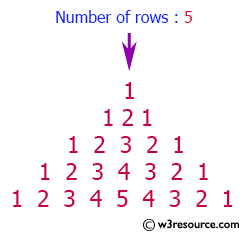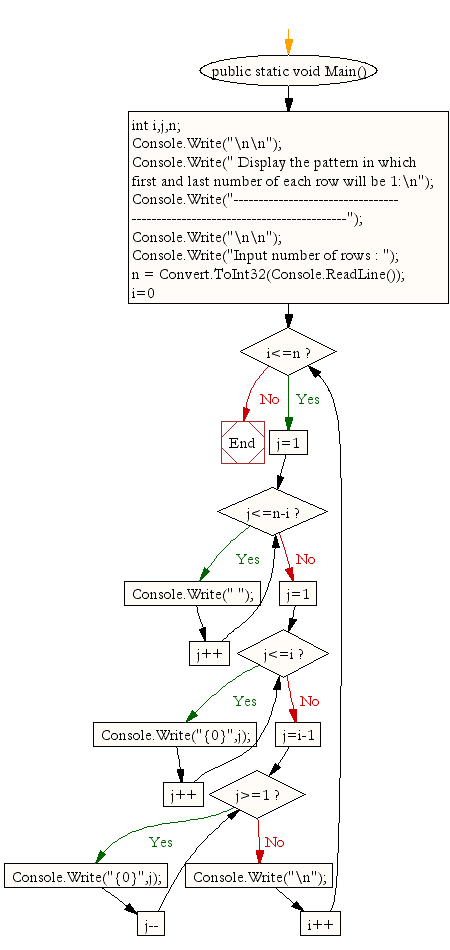﻿ C# - Pattern with 1 as the 1st and last number in each row# C# Sharp Exercises: Display the pattern in which the first and a last number of each row will be 1

## C# Sharp For Loop: Exercise-36 with Solution

Write a program in C# Sharp to display the such a pattern for n number of rows using a number which will start with the number 1 and the first and a last number of each row will be 1.
The pattern is as follows :

```  1
121
12321
```

Pictorial Presentation:Sample Solution:

C# Sharp Code:

``````using System;
public class Exercise36
{
public static void Main()
{
int i,j,n;

Console.Write("\n\n");
Console.Write(" Display the pattern in which first and last number of each row will be 1:\n");
Console.Write("----------------------------------------------------------------------------");
Console.Write("\n\n");

Console.Write("Input number of rows : ");
for(i=0;i<=n;i++)
{
/* print blank spaces */
for(j=1;j<=n-i;j++)
Console.Write(" ");
/* Display number in ascending order upto middle*/
for(j=1;j<=i;j++)
Console.Write("{0}",j);

/* Display  number in reverse order after middle */
for(j=i-1;j>=1;j--)
Console.Write("{0}",j);

Console.Write("\n");
}
}
}
```
```

Sample Output:

``` Display the pattern in which first and last number of each row will be 1:
----------------------------------------------------------------------------
Input number of rows : 5
1
121
12321
1234321
123454321
```

Flowchart:C# Sharp Code Editor:

Contribute your code and comments through Disqus.

What is the difficulty level of this exercise?

Test your Programming skills with w3resource's quiz.

﻿